## Deep Learning with Keras and TensorFlow

Certification Training
6547 Learners
7 Chapters +

# Multilayer Artificial Neural Network

Welcome to the fourth lesson, ‘Multilayer ANN’ of the Deep Learning Tutorial, which is a part of the Deep Learning (with TensorFlow) Certification Course offered by Simplilearn. This lesson gives you an overview of Multilayer ANN along with overfitting and underfitting.

Let us begin with the objectives of this lesson.

## Objectives

After completing this lesson on Multilayer ANN, you’ll be able to:

• Analyze how to regularize and minimize the cost function in a neural network

• Carry out backpropagation to adjust weights in a neural network

• Inspect convergence in a multilayer ANN

• Explore multilayer ANN

• Implement forward propagation in multilayer perceptron (MLP)

• Understand how the capacity of a model is affected by underfitting and overfitting

## Single-layer ANN - A RECAP

Perceptron rule and Adaline rule were used to train a single-layer neural network.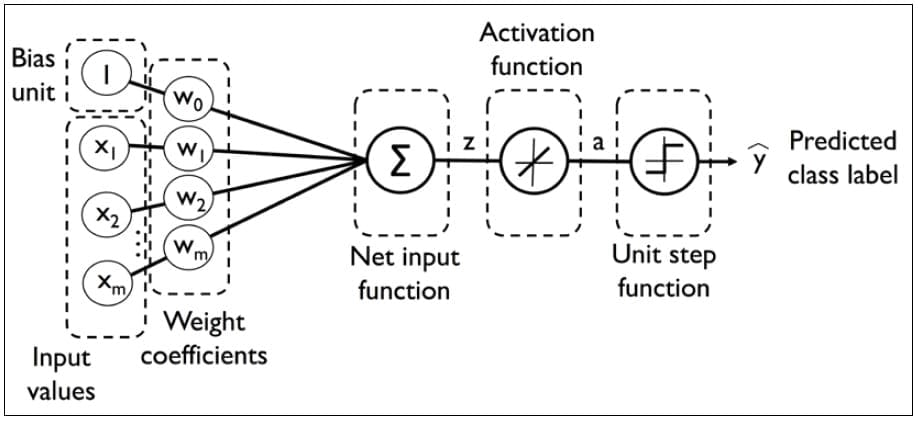Weights are updated based on a unit function in perceptron rule or on a linear function in Adaline Rule.

## History of Multi-layer ANN

Deep Learning deals with training multi-layer artificial neural networks, also called Deep Neural Networks.

After Rosenblatt perceptron was developed in the 1950s, there was a lack of interest in neural networks until 1986, when Dr.Hinton and his colleagues developed the backpropagation algorithm to train a multilayer neural network.

Today it is a hot topic with many leading firms like Google, Facebook, and Microsoft which invest heavily in applications using deep neural networks.

## Multi-layer ANN

A fully connected multi-layer neural network is called a Multilayer Perceptron (MLP).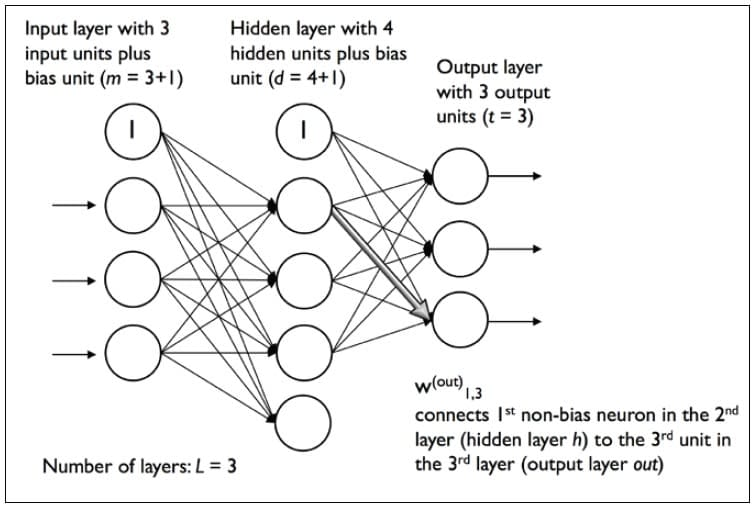It has 3 layers including one hidden layer. If it has more than 1 hidden layer, it is called a deep ANN.

An MLP is a typical example of a feedforward artificial neural network.

In this figure, the ith activation unit in the lth layer is denoted as ai(l).

The number of layers and the number of neurons are referred to as hyperparameters of a neural network, and these need tuning. Cross-validation techniques must be used to find ideal values for these.

The weight adjustment training is done via backpropagation. Deeper neural networks are better at processing data. However, deeper layers can lead to vanishing gradient problem. Special algorithms are required to solve this issue.

### Notations

In the representation below: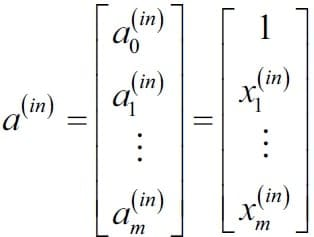• ai(in) refers to the ith value in the input layer

• ai(h) refers to the ith unit in the hidden layer

• ai(out) refers to the ith unit in the output layer

• ao(in) is simply the bias unit and is equal to 1; it will have the corresponding weight w0

• The weight coefficient from layer l to layer l+1 is represented by wk,j(l)

A simplified view of the multilayer is presented here.

This image shows a fully connected three-layer neural network with 3 input neurons and 3 output neurons. A bias term is added to the input vector.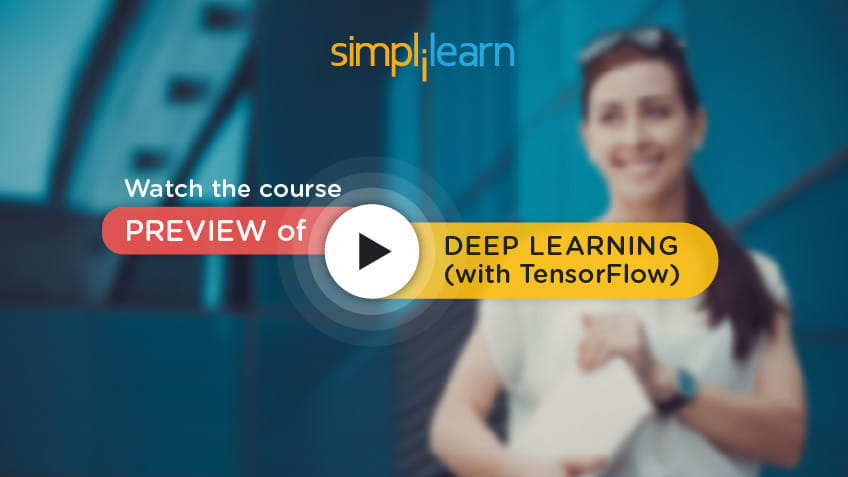## Topic 2— Forward Propagation

In the following topics, we discuss the forward propagation in detail.

### MLP Learning Procedure

The MLP learning procedure is as follows :

• Starting with the input layer, propagate data forward to the output layer. This step is the forward propagation.

• Based on the output, calculate the error (the difference between the predicted and known outcome). The error needs to be minimized.

• Backpropagate the error. Find its derivative with respect to each weight in the network, and update the model.

Repeat the three steps given above over multiple epochs to learn ideal weights.

Finally, the output is taken via a threshold function to obtain the predicted class labels.

### Forward Propagation in MLP

In the first step, calculate the activation unit al(h) of the hidden layer.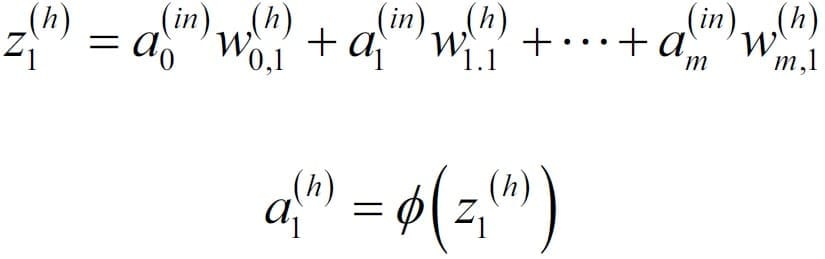Activation unit is the result of applying an activation function φ to the z value. It must be differentiable to be able to learn weights using gradient descent.

The activation function φ is often the sigmoid (logistic) function.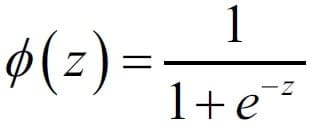It allows nonlinearity needed to solve complex problems like image processing.

### Sigmoid Curve

The sigmoid curve is an S-shaped curve.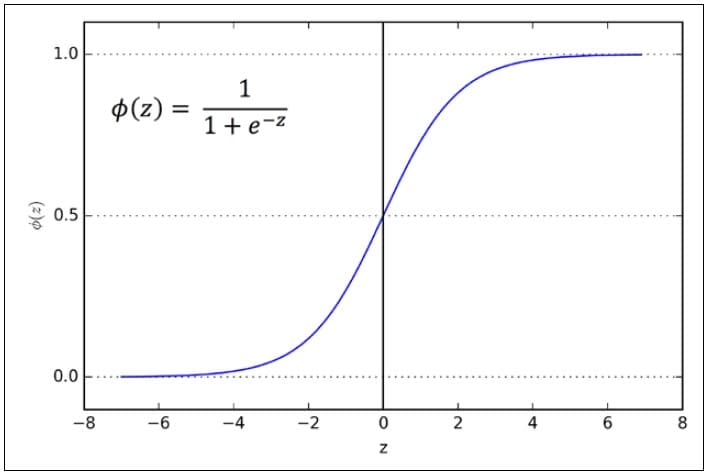### Recap of Notations

The activation of the hidden layer is represented as:

z(h) = a(in) W(h)

a(h) =

For the output layer:

Z(out) = A(h) W(out)

A(out) =

### Find our Deep Learning with Keras and TensorFlow Online Classroom training classes in top cities:

Name Date Place
Deep Learning with Keras and TensorFlow 13 Feb -7 Mar 2021, Weekend batch Your City View Details
Deep Learning with Keras and TensorFlow 13 Mar -4 Apr 2021, Weekend batch New York City View Details
Deep Learning with Keras and TensorFlow 17 Apr -9 May 2021, Weekend batch San Francisco View Details
Related Courses
Learner Reviews
Related Articles
• Disclaimer
• PMP, PMI, PMBOK, CAPM, PgMP, PfMP, ACP, PBA, RMP, SP, and OPM3 are registered marks of the Project Management Institute, Inc.

Name*
Email*
Phone Number*